# 1 Context

In order to illustrate the package SMM we introduce a practical example taken from Barbu and Limnios (2008), where a semi-Markov modeling is appropriate. Let $$\mathcal{F}$$ be a textile factory. To avoid river pollution, the factory waste is treated in the unit $$\mathcal{U}$$ before being thrown in the river $$\mathcal{R}$$. In order for the factory not to be stopped if a failure occurs in the treatment unit $$\mathcal{U}$$, the waste is stocked in a tank $$\mathcal{T}$$. If $$\mathcal{U}$$ is repaired before $$\mathcal{T}$$ is full, then the factory starts to work again normally and we suppose that the tank is emptied instantaneously (before another failure of $$\mathcal{U}$$). Otherwise, the whole factory has to stop and a certain time is necessary to restart it. The figure below describes the waste treatment system of the textile factory.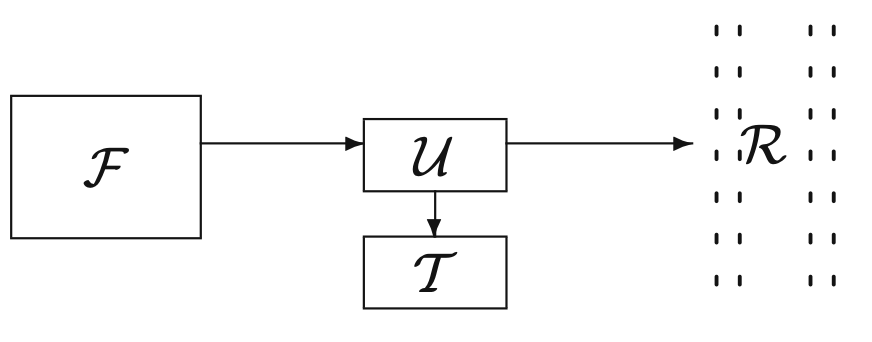Waste treatment for a textile factory

As the output of the factory is variable, the filling time of the tank is a random variable. Moreover, if we suppose that the filling time is deterministic, we can still make the assumption that we have a random time that has a Dirac distribution.

We propose a semi-Markov modelization for the evolution of the described system. Let $$E = \{\texttt{"}1\texttt{"},\ \texttt{"}2\texttt{"},\ \texttt{"}3\texttt{"}\}$$ be the set of possible states of the system, where we set:

• State 1 - everything is working, that is, $$\mathcal{F}$$ and $$\mathcal{U}$$ are in good state and $$\mathcal{T}$$ is empty;
• State 2 - $$\mathcal{U}$$ has failed, but $$\mathcal{T}$$ is not yet full, so the factory is still working;
• State 3 - $$\mathcal{U}$$ has failed and $$\mathcal{T}$$ is full, so the factory is not working.

The possible transitions between states are given in the following figure: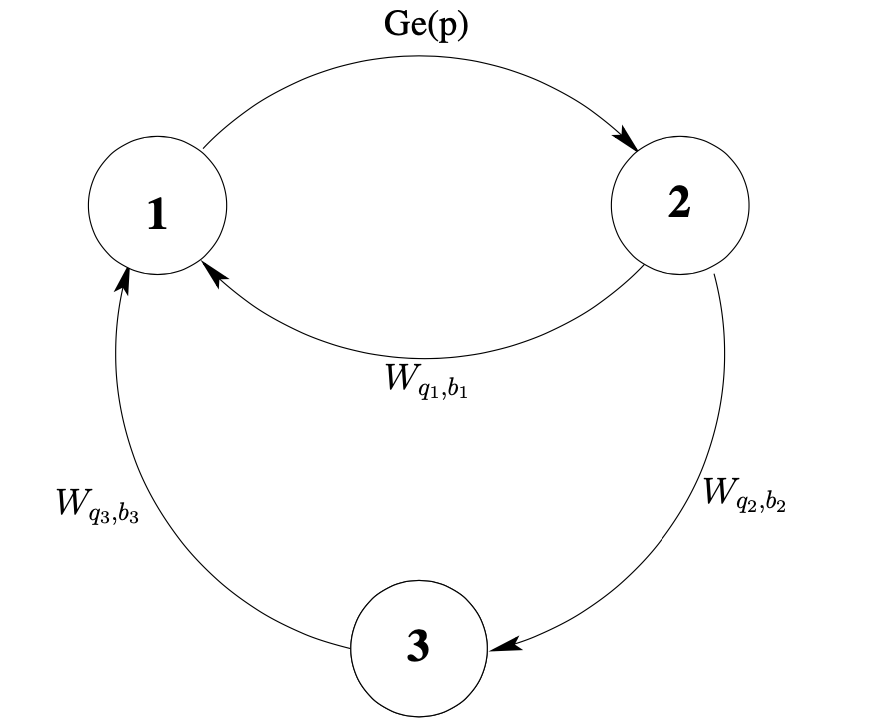Three-state discrete-time semi-Markov system modelization

The system is defined by:

• The initial distribution $$\boldsymbol{\alpha} = (\alpha_1,\ \alpha_2,\ \alpha_3)$$;

• The transition matrix $$\boldsymbol{p}$$ of the embedded Markov chain $$(J_n)_{n \in \mathbb{N}}$$ $$\boldsymbol{p} = \begin{pmatrix} 0 & 1 & 0 \\ a & 0 & b \\ 1 & 0 & 0 \end{pmatrix}$$,

• The conditional sojourn time distributions $$\boldsymbol{f(k)} = \begin{pmatrix} 0 & f_{12}(k) & 0 \\ f_{21}(k) & 0 & f_{23}(k) \\ f_{31}(k) & 0 & 0 \end{pmatrix}, \ k \in \mathbb{N}$$,

where,

• $$f_{12}$$ is the distribution of the failure time of the treatment unit $$\mathcal{U}$$;

• $$f_{21}$$ is the distribution of the repairing time of $$\mathcal{U}$$;

• $$f_{23}$$ is the distribution of the filling time of the tank $$\mathcal{T}$$;

• $$f_{31}$$ is the distribution of time needed to restart the entire factory $$\mathcal{F}$$, after it has been shut down.

This is an irreducible discrete-time semi-Markov system, or, equivalently, a Markov renewal system. We chose the following sojourn time distributions:

• $$f_{12}$$ is the geometric distribution on $$\mathbb{N}^{*}$$ of parameter $$p$$, $$0 < p < 1$$, i.e., $$f_{12}(k) = p(1 - p)^{k - 1},\ k \in \mathbb{N}^{*}$$.

• $$f_{21} = W_{q1, b1},\ f_{23} = W_{q2, b2},\ f_{31} = W_{q3, b3}$$ are discrete-time first-type Weibull distributions (Nakagawa and Osaki (1975)), defined by: $W_{q, b}(0) = 0,\ W_{q, b}(k) = q^{(k - 1)^{b}} - q^{k^{b}},\ k \in \mathbb{N}^{*}$

The choice of discrete-time Weibull distribution is motivated by its use in reliability theory, due to its flexibility in modeling failure rates. In fact, our main purpose in this document is to compute and estimate the main reliability indicators of this system.

# 2 Numerical application

Let us continue the example of the textile factory presented above. We take the initial distribution $$\boldsymbol{\alpha} = (1,\ 0,\ 0)$$, the transition matrix of the embedded Markov chain $$(J_{n})_{n \in \mathbb{N}}$$, $\boldsymbol{p} = \begin{pmatrix} 0 & 1 & 0 \\ 0.95 & 0 & 0.05 \\ 1 & 0 & 0 \end{pmatrix}$ and the following conditional sojourn time distributions:

• $$f_{12}$$ is the geometric distribution on $$\mathbb{N}^{*}$$ with parameter $$p = 0.8$$;

• $$f_{21} = W_{q1, b1},\ f_{23} = W_{q2, b2},\ f_{31} = W_{q3, b3}$$ are discrete-time Weibull distributions with parameters: $q_{1} = 0.3,\ b_{1} = 0.5,\ q_{2} = 0.5,\ b_{2} = 0.7,\ q_{3} = 0.6,\ b_{3} = 0.9.$

## 2.1 Specification and simulation

First, let us create a smmparametric object to represent the semi-Markov chain associated to the system:

states <- c("1", "2", "3") # State space

alpha <- c(1, 0, 0) # Initial distribution

p <- matrix(data = c(0, 1, 0,
0.95, 0, 0.05,
1, 0, 0), nrow = 3, byrow = TRUE) # Transition matrix

distr <- matrix(c(NA, "geom", NA,
"dweibull", NA, "dweibull",
"dweibull", NA, NA),
nrow = 3, ncol = 3, byrow = TRUE) # Distribution matrix

param1 <- matrix(c(NA, 0.8, NA,
0.3, NA, 0.5,
0.6, NA, NA),
nrow = 3, ncol = 3, byrow = TRUE)

param2 <- matrix(c(NA, NA, NA,
0.5, NA, 0.7,
0.9, NA, NA),
nrow = 3, ncol = 3, byrow = TRUE)

parameters <- array(c(param1, param2), c(3, 3, 2))

factory <- smmparametric(states = states, init = alpha, ptrans = p,
type.sojourn = "fij", distr = distr, param = parameters)

After that, we are able to simulate a sequence of sample sizes $$M = 10,000$$:

M <- 10000
seq <- simulate(object = factory, nsim = M)

## 2.2 Estimation

Thanks to the SMM package, we can estimate any semi-Markov model with one or several discrete sequences. In our case, we are going to introduce a non-parametric estimation:

estimate <- fitsmm(sequences = seq, states = states, type.sojourn = "fij")
## Some transitions from state i to state j are not observed: (i = "3" to j = "2"), (i = "1" to j = "3")
## The probabilities of the initial state(s) "2", "3" are 0.

The estimate $$\hat{p}$$ of the transition matrix $$p$$ is:

print(x = estimate$ptrans, digits = 2) ## 1 2 3 ## 1 0.00 1 0.00 ## 2 0.95 0 0.05 ## 3 1.00 0 0.00 We can also plot the estimated sojourn time densities. For example, let us plot the sojourn time distribution $$\hat{f}_{23}$$: plot(x = estimate, i = "2", j = "3", type = "l", col = "blue") lines(x = 1:estimate$kmax, y = ddweibull(x = 1:estimate\$kmax, q = 0.5, beta = 0.7),
col = "red", pch = "x")

legend(x = "topright",
legend = c("True value", "Estimate"),
col = c("red", "blue"), lty = c(1, 1))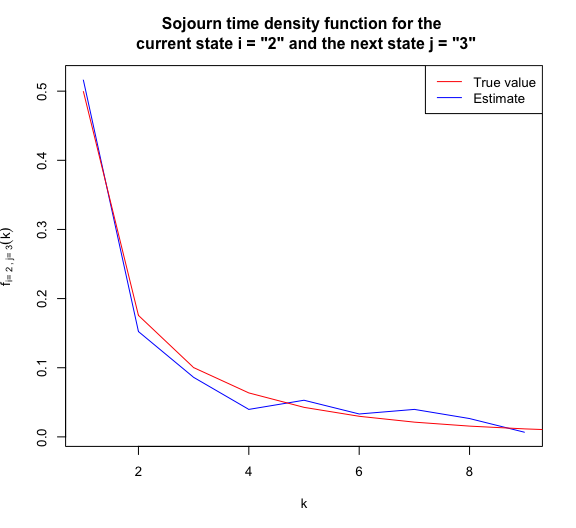In the following sections, based on the model specification factory and the estimate of the latter estimate, we can compute true and estimated values of some indicators such as reliability, availability, maintainability as well as their asymptotic confidence intervals.

## 2.3 Reliability

Consider a system $$S_{ystem}$$ starting to function at time $$k = 0$$. The reliability of $$S_{ystem}$$ at time $$k \in \mathbb{N}$$ is the probability that the system has functioned without failure in the period $$[0, k]$$. Let’s take $$k = 300$$.

k <- 300
upstates <- c("1", "2") # Working states of the semi-Markov system
trueReliab <- reliability(x = factory, k = k, upstates = upstates)
estReliab <- reliability(x = estimate, k = k, upstates = upstates)
plot(x = 0:k, y = trueReliab[, 1], type = "l", cex = 2.5, ylim = c(0, 1),
col = "red", main = "Reliability", xlab = "k", ylab = "R(k)")

lines(x = estReliab[, 1], col = "blue")
lines(x = estReliab[, 3], lty = 4, col = "blue")
lines(x = estReliab[, 4], lty = 4, col = "blue")
legend(x = "topright",
legend = c("True value", "Estimated value", "95% confidence interval"),
col = c("red", "blue", "blue"), lty = c(1, 1, 4))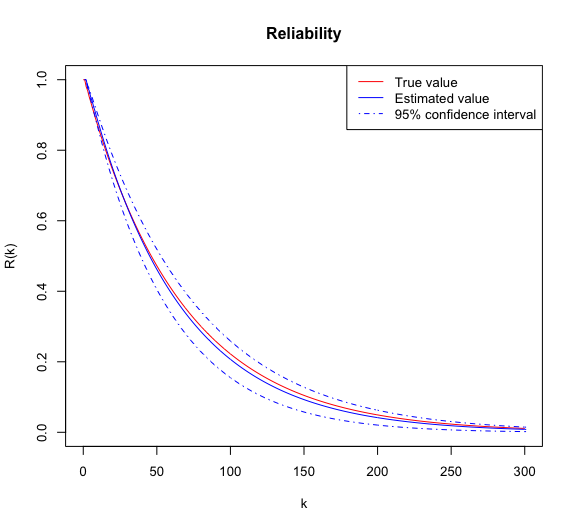## 2.4 Availability

The pointwise (or instantaneous) availability of a system $$S_{ystem}$$ at time $$k \in \mathbb{N}$$ is the probability that the system is operational at time $$k$$ (independently of the fact that the system has failed or not in $$[0, k)$$).

trueAvail <- availability(x = factory, k = k, upstates = upstates)
estAvail <- availability(x = estimate, k = k, upstates = upstates)
plot(x = 0:k, y = trueAvail[, 1], type = "l", cex = 2.5, ylim = c(0.95, 1),
col = "red", main = "Availability", xlab = "k", ylab = "A(k)")

lines(x = estAvail[, 1], col = "blue")
lines(x = estAvail[, 3], lty = 4, col = "blue")
lines(x = estAvail[, 4], lty = 4, col = "blue")
legend(x = "topright",
legend = c("True value", "Estimated value", "95% confidence interval"),
col = c("red", "blue", "blue"), lty = c(1, 1, 4))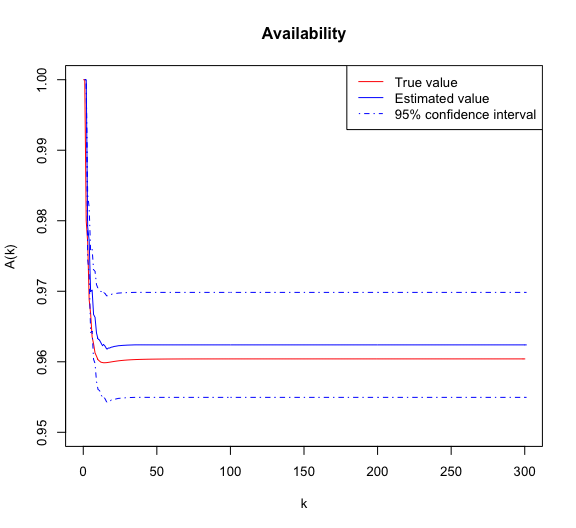## 2.5 Failure Rates

Following the lines of Barbu and Limnios (2008), we consider two different definitions of the failure rate function. The first one is the usual failure rate, introduced by Barlow, Marshall and Prochan (Barlow, Marshall, and Proschan (1963)), that we will call BMP-failure rate. The second one is a failure rate adapted for the work in discrete time, proposed by Roy and Gupta (Roy and Gupta (1992)), that we will call RG-failure rate.

### 2.5.1 BMP-failure rate

Consider a system $$S_{ystem}$$ starting to work at time $$k = 0$$. The BMP-failure rate at time $$k \in \mathbb{N},$$ denoted by $$\lambda(k),$$ is the conditional probability that the failure of the system occurs at time $$k$$, given that the system has worked until time $$k - 1$$.

trueBMP <- failureRate(x = factory, k = k, upstates = upstates)
estBMP <- failureRate(x = estimate, k = k, upstates = upstates)
plot(x = 0:k, y =  trueBMP[, 1], type = "l", cex = 2.5, ylim = c(0, 0.025),
col = "red", main = "BMP-failure rate", xlab = "k", ylab = bquote(lambda(k)))

lines(x = estBMP[, 1], col = "blue")
lines(x = estBMP[, 3], lty = 4, col = "blue")
lines(x = estBMP[, 4], lty = 4, col = "blue")
legend(x = "topright",
legend = c("True value", "Estimated value", "95% confidence interval"),
col = c("red", "blue", "blue"), lty = c(1, 1, 4))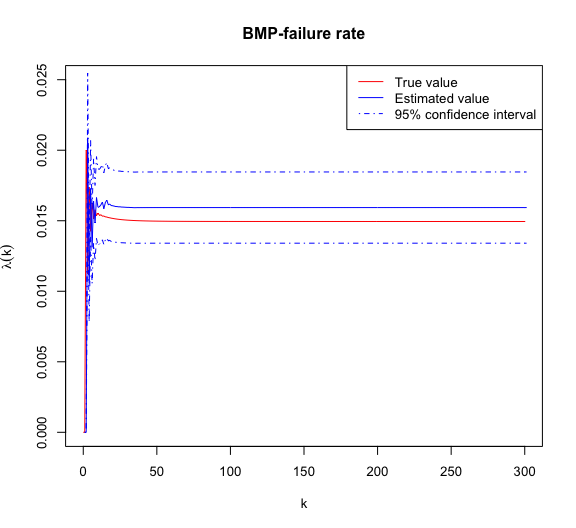### 2.5.2 RG-failure rate

The RG-failure rate will be denoted by $$r(k),\ k \in \mathbb{N}$$.

trueRG <- failureRate(x = factory, k = k, upstates = upstates, failure.rate = "RG")
estRG <- failureRate(x = estimate, k = k, upstates = upstates, failure.rate = "RG")
plot(x = 0:k, y =  trueRG[, 1], type = "l", cex = 2.5, ylim = c(0, 0.03),
col = "red", main = "RG-failure rate", xlab = "k", ylab = "r(k)")

lines(x = estRG[, 1], col = "blue")
lines(x = estRG[, 3], lty = 4, col = "blue")
lines(x = estRG[, 4], lty = 4, col = "blue")
legend(x = "topright",
legend = c("True value", "Estimated value", "95% confidence interval"),
col = c("red", "blue", "blue"), lty = c(1, 1, 4))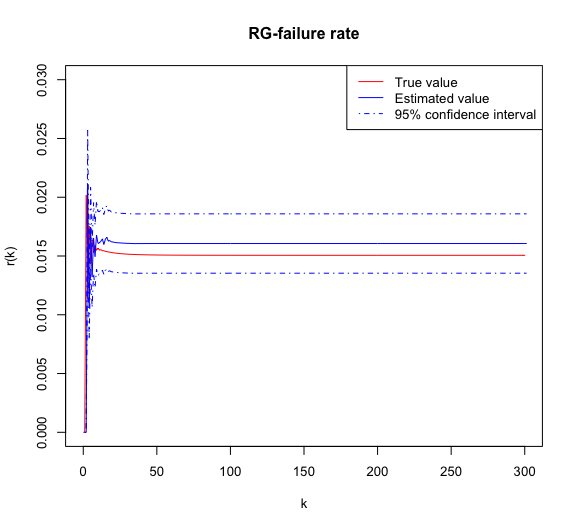## 2.6 Mean Times

### 2.6.1 Mean time to failure (MTTF)

Consider a system $$S_{ystem}$$ starting to work at time $$k = 0$$. The mean time to failure (MTTF) is defined as the mean lifetime.

trueMTTF <- mttf(x = factory, upstates = upstates)
estMTTF <- mttf(x = estimate, upstates = upstates)
print(trueMTTF)
##       mttf   sigma2
## 1 67.19099 307414.6
## 2 65.94099 307372.0
print(estMTTF)
##       mttf   sigma2      lwr    upper
## 1 63.72848 256517.4 53.80174 73.65521
## 2 62.48324 256478.9 52.55725 72.40923

### 2.6.2 Mean time to repair (MTTR)

Consider a system $$S_{ystem}$$ that has just entered the failure states at time $$k = 0$$. The mean time to repair (MTTR) is defined as the mean of the repair duration.

trueMTTR <- mttr(x = factory, upstates = upstates)
estMTTR <- mttr(x = estimate, upstates = upstates)